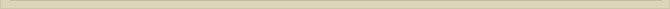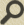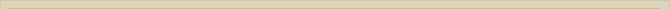## The Quantum Distance and the Second Quantum Dimension (Compton-radius)

When you are interested in physics you must read “Unbelievable“!

The Planck-radius is the smallest known distance so we assume that the quantum distance is: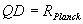We have seen that the ratio between the classical radius of the electron, the Compton radius (Rc), and QD is the same as the ratio between the rydberg-distance Rr and the Bohr-distance. Is this a coincidence or not?

We assume the ratio has the integer value of: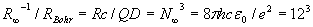In figure 3 the quantum numbers 6 and 12 already give some symmetry. First we will concentrate on the quantum number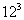and show that with this number we can create “homogeneous” space.

In figure 4 schematically the Compton-radius is the radius of the drawn circle.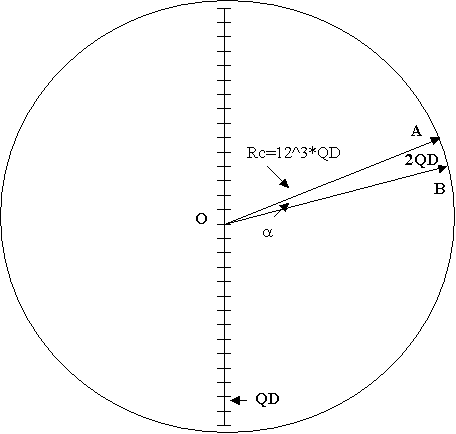Figure 4. The quantum distance transformation.

Calculation gives 25 minutes for the angle α (=Alpha=Fine Structure Constant=2π/864***) in figure 4. In 360 degrees there are exact 864 angles of 25 minutes. The perimeter of the “circle” in figure 4, the sum of all 864 straight lines AB=2*QD, is: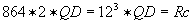The perimeter of the created circle is Rc (864 angles α of 25’=360 degrees) while the perimeter of a circle in the macro-world is 2πRc !

This result is remarkable. How can Rc be 2πRc at the same time?

When we want to compare the quantum perimeter with the macro-world perimeter the correction factor is 2π.

The straight line AB, the basis of triangle OAB, is 2*QD. The surface of one triangle OAB is: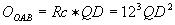The total surface of one side with 864 triangles is: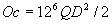.

The surface of both sides of the created “circle” hastriangles with a total surface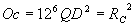.

The “macro-world” surface of two circles with radius Rc is Oc=2πRc^2, so with the surface there is also a “translation” factor of 2π for the transformation from the QD to Rc level.Figure 5. Illustration of the imperfect Quantum Space at the Compton-distance.

At the Compton-level Rc/2 point-volumes create a “perfect” circle for observers in O (figure 4). The observer in O can observe no more than two, right angled “perfect” circles, at the same time at distance Rc.  Because there is no restriction for the angle of observation of the two “perfect” circles, one should be able to observe the circles in “any” direction, but not at the same time (the point-volumes create at Rc the 2-dimensional quantum space).

The quantum bulbs at Rc (figure 5) touch each other in such a way that they can form with/2 QD-bulbs a “perfect” circle around O; all QD-bulbs of circle Rc are “in touch”. One can observe that the QD-bulbs up and down Rc (figure 5) do not have closed perimeters because “curved” 3-dimensional space around a charge cannot be filled continuously with bulbs. Inhomogeneity is unavoidable.

Next chapter: The Transformation to the third Quantum Dimension (Bohr-distance)